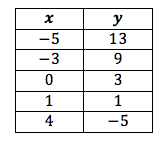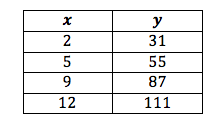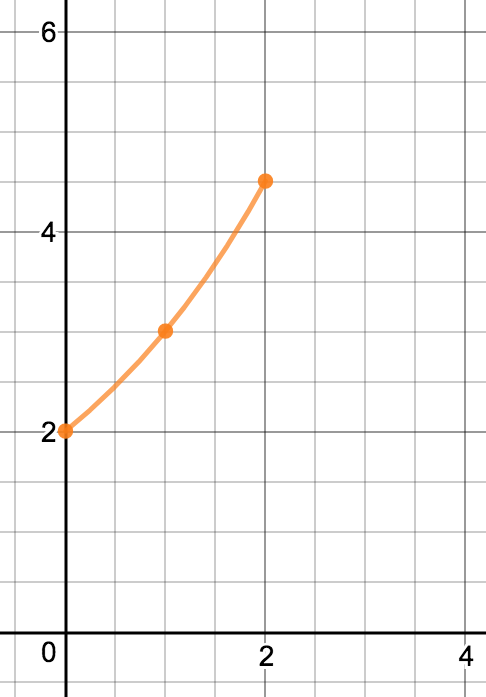# Linear Equations, Inequalities and Systems

## Objective

Determine if a function is linear based on the rate of change of points in the function presented graphically and in a table of values.

## Common Core Standards

### Core Standards

?

• F.IF.B.6 — Calculate and interpret the average rate of change of a function (presented symbolically or as a table) over a specified interval. Estimate the rate of change from a graph. Modeling is best interpreted not as a collection of isolated topics but in relation to other standards. Making mathematical models is a Standard for Mathematical Practice, and specific modeling standards appear throughout the high school standards indicated by a star symbol (★). The star symbol sometimes appears on the heading for a group of standards; in that case, it should be understood to apply to all standards in that group.

• F.IF.C.7.A — Graph linear and quadratic functions and show intercepts, maxima, and minima.

• F.IF.C.9 — Compare properties of two functions each represented in a different way (algebraically, graphically, numerically in tables, or by verbal descriptions). For example, given a graph of one quadratic function and an algebraic expression for another, say which has the larger maximum.

• F.LE.A.1.A — Prove that linear functions grow by equal differences over equal intervals, and that exponential functions grow by equal factors over equal intervals.

?

• 8.F.A.2

• 8.F.A.3

• 8.F.B.4

• 8.F.B.5

## Criteria for Success

?

1. Given a table of values, identify the rate of change between two points.
2. Describe the difference between subsequent  $y$-values, given equal intervals on the $x$-values.
3. Write an equation from a table of values.
4. Compare a table of values with a graph of a linear equation, and describe which has the larger rate of change.
5. Identify graphs that appear linear but based on the non-constant rate of change are not linear.

## Anchor Problems

?

### Problem 1

The following table of values shows some solutions to a linear equation.

Write the linear equation that contains these solutions.### Problem 2

Jonas wants to borrow money from his parents to buy a phone and has two options for a payment schedule: one presented in a table and one presented as an equation.

Payment Schedule A:Payment Schedule B:  ${4x + 25 = y}$ where $y$ is cost of the phone, and $x$ is the number of weeks required to pay Jonas’s parents back.

Which one has the greater rate of change? What does this mean in context?

### Problem 3

Describe why this graph is not linear.